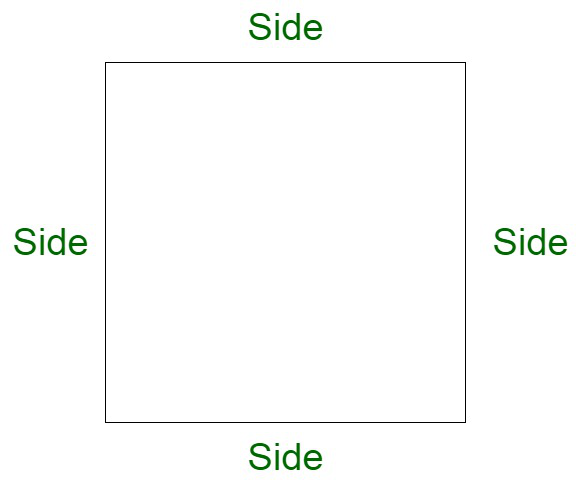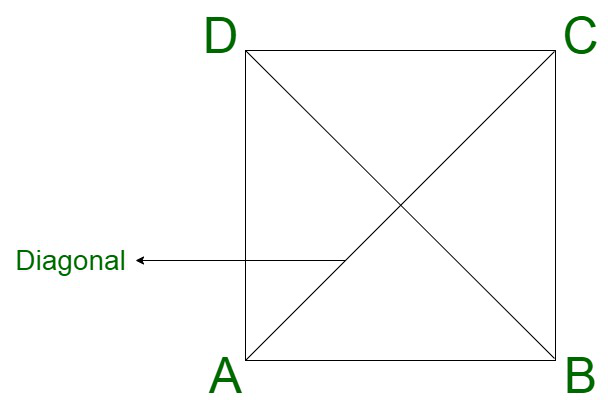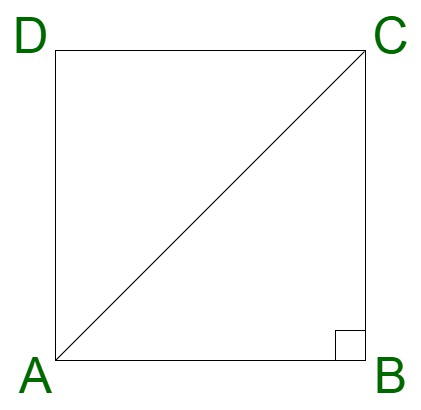# What is the length of the diagonal of a square whose area is 16900m2?

• Last Updated : 21 Nov, 2021

Square is a two-dimensional figure which is associated with four equal and parallel sides. All the sides are equal in length. All the angles corresponding to the corners of the square are equal to right angles.

A square can be considered as a quadrilateral associated with the following properties:

• the opposite sides are parallel.
• all four sides are equal.
• all angles measure 90°.### Area of Square

The area is the space enclosed within the boundaries of the object. The area of a square can be measured with the length of any of the sides of the square. All the sides of a square are equal and therefore, its final area is equivalent to the square of the side.

Area of the square using sides;

Area of square = side × side = side2

### Diagonal of a Square

A square contains two diagonals which are formed by joining the opposite two sides of the square. The diagonals of the square are associated with the following set of properties :

• The diagonals of a square are equal in length.
• They are perpendicular bisectors of each other.
• Diagonals divide it into two congruent isosceles right-angled triangles.

### Area of square using diagonals

Let us consider a square of side s. Since all the sides are equal, therefore, each side is equivalent to s.

Now,

In triangle ABC,

AB = s

BC =s

Let us assume diagonal, AC to be d.

Now, In Pythagoras theorem,

Diagonal AC2 = AB2 + BC2   (By Pythagoras theorem)

AC =  √(AB2 + BC2)

AC = √(s2 + s2)

AC = √2s

Now, Area = 1/2 × d2,  where d is the diagonal of the square.### What is the length of the diagonal of a square whose area is 16900 m2?

Solution:Area of square = side × side

Assume the side of the square be ‘s’

Area of square = s2

Here area of the square is given 16900 m2

Putting the value of the area in the formula

16900 = s2

s = √16900

s = 130 m

Thus,

Side of the square is 130 m

Now finding the diagonal of the square using its side

Diagonal AC2 = AB2 + BC2   (By Pythagoras theorem)

AC =  √(AB2 + BC2)

AC = √(1302 + 1302)

AC = 130√2 m

Therefore,

The length of the diagonal of the square of area 16900 m2 is 130√2 m.

### Sample Questions

Question 1. If the diagonal of a square is 60 cm, then find the area of the square?

Solution:

Here we have to find the area of the square using the given diagonal,

We are given that the diagonal of the square is 60 cm

As we know that

Area of square = 1/2 d2

Here d is the diagonal

Area of the square = 1/2 × d2

Area of the square = 1/2 × 60 × 60

Area of the square = 1800 cm2

Therefore,

Area of the square with a diagonal of 60 cm is 1800 cm2.

Question 2. Find the diagonal of the square if its area is 2500 m2?

Solution:

Here we have to find the diagonal of the square whose area is given

Given the area of the square is 2500 m2

As we know that

Area of the square = Side × Side

Assume the side of the square be ‘s’

Area of the square = s2

2500 = s2

s = √2500

s = 50 cm

Further,

As we know that

Diagonal of the square = side√2

Diagonal of the square = s√2

Diagonal of the square = 50√2 cm

Therefore,

The diagonal of the square with the area 2500 cm2 is 50√2 cm.

Question 3. Find the perimeter of the square with the diagonal 100 cm?

Solution:

Here we have to find the perimeter of the square whose diagonal is given

Here we have

Diagonal = 100 cm

As we know that

Diagonal of the square = side√2

Assume the side of the square be ‘s’

100 = s√2

s = 100/√2 cm

Now,

Perimeter of the square = 4 × side

Perimeter of the square = 4 × s

Perimeter of the square = 4 × 100/√2

Perimeter of the square = 400/√2 cm

Therefore,

Perimeter of the square is 400/√2.

Question 4. If the diagonal of a square park is 75√2 m then find the cost of fencing the square park at the rate of ₹50 per meter?

Solution:

Here we have to find the cost of fencing the square park

As we know that

Diagonal of square = side√2

Assume the side of the square park is ‘s’

75√2 = s√2

s = 75 m

Thus,

Side of the square park is 75 m

Now,

Finding the cost of fencing

Perimeter of square = 4 × side

Perimeter of square = 4 × 75

Perimeter of square = 300 m

Cost of fencing = ₹50 × 300

Cost of fencing = ₹15000

Therefore,

Cost of fencing square park is ₹15000.

Question 5. Find the area of the square with a diagonal of 120 cm?

Solution:

Here we have to find the area of the square using the given diagonal,

We are given that the diagonal of the square is 120 cm

As we know that

Area of square = 1/2 d2

Here d is the diagonal

Area of the square = 1/2 × d2

Area of the square = 1/2 × 120 × 120

Area of the square = 7200 cm2

Therefore,

Area of the square with a diagonal of 120 cm is 7200 cm2.

My Personal Notes arrow_drop_up